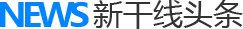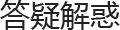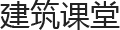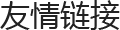让工作变得顺心更多头条>进入栏目>

行家精彩回答进入栏目>

课堂首发• 北京
• 天津
• 上海
• 重庆

A,F,G,H

• 安徽
• 福建
• 甘肃
• 广东
• 广西
• 贵州
• 海南
• 河北
• 河南
• 湖北
• 湖南
• 黑龙江

J,L,N,Q

• 吉林
• 江苏
• 江西
• 辽宁
• 宁夏
• 内蒙古
• 青海

S,X,Y,Z

• 山东
• 山西
• 陕西
• 四川
• 西藏
• 新疆
• 云南
• 浙江

• 昌平